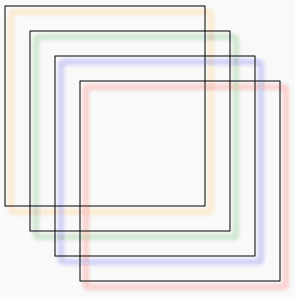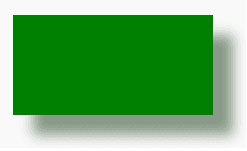In HTML5 canvas, you can add shadows on a shape, a line, text, or an image which can create a sense of third dimension. To add shadows with the HTML5 Canvas, you can use the following properties of the canvas context.

The property is used to get or set the horizontal distance of a shadow from a shape. You can use positive or negative values to control the position of a shadow. The default value is zero.

Syntax:

`ctx.shadowOffsetX = h_distance;`

Where h_distance (type: number) is the horizontal distance of a shadow from a shape.

The property is used to get or set the vertical distance of a shadow from a shape. You can use positive or negative values to control the position of a shadow. The default value is zero.

Syntax:

`ctx.shadowOffsetX = v_distance;`

Where v_distance (type: number) is the vertical distance of a shadow from a shape.

The property is used to get or set the color to use for shadows.

Syntax:

`ctx.shadowColor`

Where shadowColor (type: string) is the CSS color.

The property is used to get or set the current level of blur that is applied to shadows.

Syntax:

`ctx.shadowBlur = blur_value`

Where blur_value is the amount of blur (type: number) that is applied to shadows.

The following web document creates a series of squares that have different degrees of shadow blur.

Output:Code:

``````<!DOCTYPE html>
<html>
<body>
<canvas id="DemoCanvas" width="500" height="600"></canvas>
<script>
var canvas = document.getElementById("DemoCanvas");
if (canvas.getContext)
{
var ctx = canvas.getContext('2d');
ctx.strokeRect(25, 25, 200, 200);
ctx.strokeRect(50, 50, 200, 200);
ctx.strokeRect(75, 75, 200, 200);
ctx.strokeRect(100, 100, 200, 200);
}
</script>
</body>
</html>``````

Here is an another example :

Output:Code:

``````<!DOCTYPE html>
<html>
<body>
<canvas id="DemoCanvas" width="500" height="600"></canvas>
<script>
var canvas = document.getElementById("DemoCanvas");
if (canvas.getContext)
{
var ctx = canvas.getContext('2d');
ctx.rect(100, 40, 200, 100);
ctx.fillStyle = 'green';
ctx.fill();
}
</script>
</body>
</html>``````

The following web document creates shadow on text.

Output:Code :

``````<!DOCTYPE html>
<html>
<body>
<canvas id="DemoCanvas" width="500" height="600"></canvas>
<script>
var canvas = document.getElementById("DemoCanvas");
if (canvas.getContext)
{
var ctx = canvas.getContext('2d');
ctx.fillStyle = 'green';
//ctx.fill();
ctx.font = 'italic 32px sans-serif';
ctx.fillText('HTML5 Canvas Tutorial', 10, 50);

ctx.fillStyle = 'red';